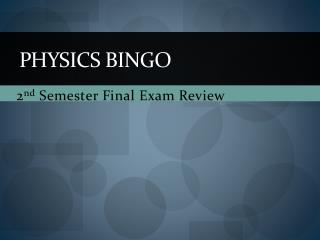DownloadDownload PresentationPhysics Bingo

# Physics Bingo

Download Presentation## Physics Bingo

- - - - - - - - - - - - - - - - - - - - - - - - - - - E N D - - - - - - - - - - - - - - - - - - - - - - - - - - -
##### Presentation Transcript

1. Physics Bingo 2nd Semester Final Exam Review

2. Rules for Bingo • Answer the problem presented. • Locate and mark the corresponding answer on your board. • You must get 5 across, down or diagonal to win. • 1st person to call BINGO each round will win some candy!

3. Problem #1 • Acceleration is a change in this…..

4. Problem #2 • A car starts from rest and reaches 33m/s in 11 seconds. • Calculate the acceleration.

5. PROBLEM #3 • If you were given a v-t graph, you would need to calculate this to find acceleration.

6. PROBLEM #4 • TheONLY force that acts on a projectile is……

7. PROBLEM #5 • If an object has a Vx of 5m/s and is in the air for 7 seconds, this is the horizontal range of the projectile.

8. PROBLEM #6 • What type of force is also the weight of the object?

9. PROBLEM #7 Which of Newton’s laws states that for every action there is an equal but opposite reaction.

10. PROBLEM #8 • Two objects (A and B) of the same mass are pushed. Object A is pushed with 15N of force and Object B is pushed with 6N. Which experiences more acceleration?

11. PROBLEM #9 • This type of energy exists when friction or an impact occurs.

12. PROBLEM #10 • What is the unit for energy?

13. PROBLEM #11 • According to the 2nd law, if you increase the mass of an object and keep the Fnet the same, the acceleration ___________________.

14. PROBLEM #12 • The “k-value” in the elastic energy equation tells you about the ___________ of the spring.

15. PROBLEM #13 • A pencil rolls off a student’s desk. It takes 0.4 seconds for it to hit the ground. What is the height of the desk?

16. PROBLEM #14 • Calculate the kinetic energy of a stock car that has a mass of 1545kg and is moving with a velocity of 57 m/s.

17. PROBLEM #15 • The slope on a _____-time graph will give you the velocity of the object.

18. PROBLEM #16 • The last two-digits of the year Mrs. Henk graduated high school and the number for her favorite NASCAR driver is ___________.

19. PROBLEM #17 • If an object is going forward and slowing down, this is the sign for the acceleration.

20. PROBLEM #18 • Lance Armstrong is training for the Tour de France. He accelerates his bike from rest to 50 m/s in 13 seconds. • Determine his displacement during this time.

21. PROBLEM #19 • A high school track and field athlete prepares to throw a shot put. If the mass of the shot is 5.45 kg. • If the athlete applies 16N of force on the shot put ball, how much acceleration does it experience?

22. PROBLELM #20 • This type of force is often referred to as a “support force.” • It is always perpendicular to the surface that the object is resting on.

23. PROBLEM #21 • At the tallest spot on a roller coaster, the riders experience the most of this type of energy.

24. PROBLEM #22 • Calculate the gravitational energy for a 57kg passenger on a plane that is 10,500 meters off of the ground.

25. PROBLEM #23 • This is the only type of energy that we studied that does not have its own specific equation.

26. PROBLEM #24 • A baseball is hit upward. If it reaches a height (d) of 14m, how much time is it in the air?

27. PROBLEM #25 • Which of Newton’s laws states that “objects in motion and objects at rest will stay in the same state unless they experience a net force greater than zero?

28. PROBLEM # 26 • A ______ is a push or a pull.

29. PROBLEM #27 • The trig function that you use when the side you are solving for is adjacent to the angle is….

30. PROBLEM #28 • The 1st car Mrs. Henk owned was a ___________.

31. PROBLEM #29 • When a string, rope or wire is stretched, the type of force is called ____________.

32. PROBLEM #30 • The law that allows us to calculate the amount of force between charges is called…